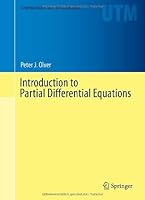# Introduction to Partial Differential Equations1 Reviews
2013-11-20
650 pages

## Book Description

This textbook is designed for a one year course covering the fundamentals of partial differential equations, geared towards advanced undergraduates and beginning graduate students in , science, engineering, and elsewhere. The exposition carefully balances solution techniques, rigor, and significant applications, all illustrated by numerous examples. Extensive exercise sets appear at the end of almost every subsection, and include straightforward computational problems to develop and reinforce new techniques and results, details on theoretical developments and proofs, challenging projects both computational and conceptual, and supplementary material that motivates the student to delve further into the subject.

No previous experience with the subject of partial differential equations or Fourier theory is assumed, the main prerequisites being undergraduate , both one- and multi-variable, ordinary differential equations, and basic linear algebra. While the classical topics of separation of variables, , boundary value problems, Green's functions, and special functions continue to form the core of an introductory course, the inclusion of nonlinear equations, shock wave dynamics, symmetry and similarity, the Maximum Principle, financial models, dispersion and solitons, Huygens'.

Principle, mechanical systems, and more make this text well attuned to recent developments and trends in this active field of contemporary research. Numerical approximation schemes are an important component of any introductory course, and the text covers the two most basic approaches: finite differences and finite elements.

Peter J. Olver is professor of mathematics at the University of Minnesota. His wide-ranging research interests are centered on the development of symmetry-based methods for differential equations and their manifold applications. He is the author of over 130 papers published in major scientific research journals as well as 4 other books, including the definitive Springer graduate text, Applications of Lie Groups to Differential Equations, and another undergraduate text, Applied Linear Algebra.

Chapter 1 What Are Partial Differential Equations?
Chapter 2 Linear and Nonlinear Waves
Chapter 3 Fourier Series
Chapter 4 Separation of Variables
Chapter 5 Finite Differences
Chapter 6 Generalized Functions and Green’s Functions
Chapter 7 Fourier Transforms
Chapter 8 Linear and Nonlinear Evolution Equations
Chapter 9 A General Framework for Linear Partial Differential Equations
Chapter 10 Finite Elements and Weak Solutions
Chapter 11 Dynamics of Planar Media
Chapter 12 Partial Differential Equations in Space

Appendix A Complex Numbers
Appendix B Linear Algebra

## Book Details

• Title: Introduction to Partial Differential Equations
• Author:
• Length: 650 pages
• Edition: 2014
• Language: English
• Publisher:
• Publication Date: 2013-11-20
• ISBN-10: 3319020986
• ISBN-13: 9783319020983